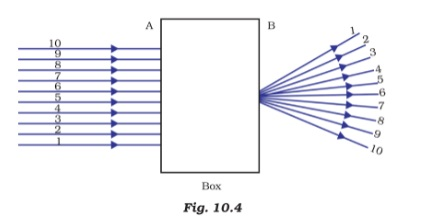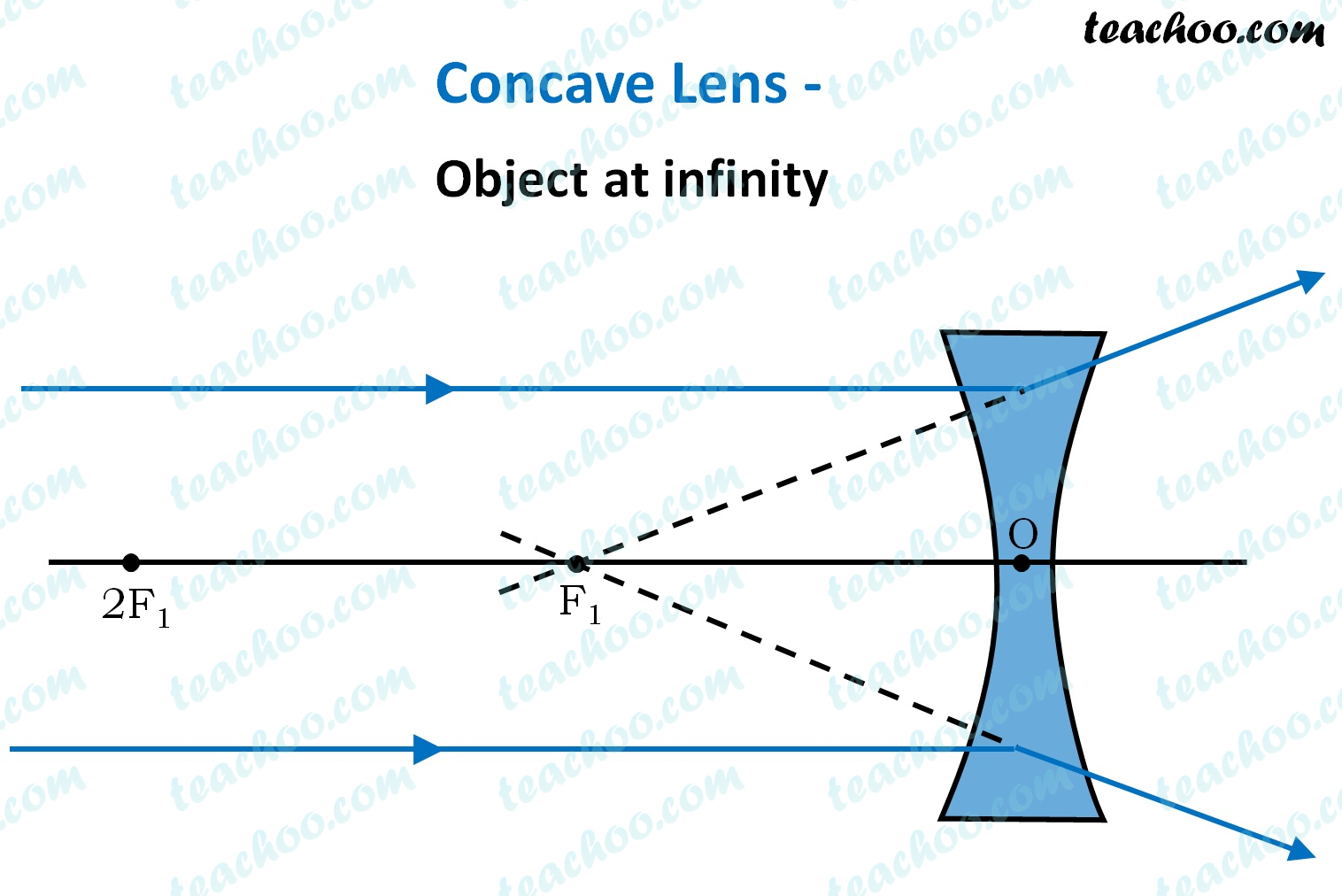MCQs from NCERT Exemplar

Class 10
Chapter 10 Class 10 - Light - Reflection and Refraction

## A beam of light is incident through the holes on side A and emerges out of the holes on the other face of the box as shown in the Figure 10.4. Which of the following could be inside the box?## (d) Convex lens

Let us go through the options.

(a) A concave lens : The incident beams of light are parallel. A concave lens would make parallel rays diverge .   The given figure is consistent with the behaviour of light passing through a Concave lens.• The emergent beams that come out from C and D are laterally shifted and parallel to the incident rays. which is not the case here

(c) A prism : A prism splits white light into 7 colours which emerge at an angle after diverging from their original paths. That is not the case here .

(d) A convex lens :  The incident rays passing through the holes A and B are parallel. A convex lens makes parallel rays converge onto a single point (the focus), which is also not the case here

So, the correct answer is (a)

Get live Maths 1-on-1 Classs - Class 6 to 12